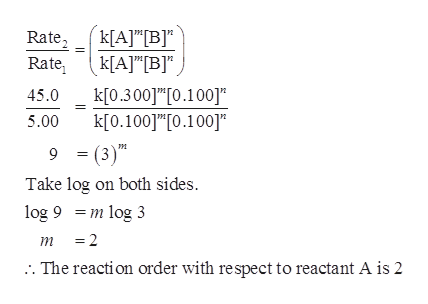# For the reaction 4A(g) + 3B(g)→2C(g) The following data were obtained at constant temperature: ExperimentInitial [A](mol/L)Initial [B](mol/L)Initial Rate(mol/L·min)10.1000.100520.3000.1004530.1000.2001040.3000.20090Determine the reaction order with respect to each reactant. Reaction order for A:     Reaction order for B:

Question
197 views

For the reaction

4A(g) + 3B(g)

2C(g)

The following data were obtained at constant temperature:

 Experiment Initial [A] (mol/L) Initial [B] (mol/L) Initial Rate (mol/L · min) 1 0.100 0.100 5 2 0.300 0.100 45 3 0.100 0.200 10 4 0.300 0.200 90

Determine the reaction order with respect to each reactant.

 Reaction order for A: Reaction order for B:

check_circle

Step 1

Consider order of reaction w.r.t [A] as m and order of reaction w.r.t [B] as n.

The rate expression would be,

Rate = k[A]m[B]n

Step 2

Order with respect to reactant A:

From the given data, identify two reactions in which...help_outlineImage Transcriptionclosek[A]T[B]" k[AJ"[B]" Rate Rate k[0.300]1"[0.100]" 45.0 k[0.100"[0.100] 9 (3) Take log on both sides 5.00 т log 9m log 3 =2 т . The reaction order with respect to reactant A is 2 fullscreen

### Want to see the full answer?

See Solution

#### Want to see this answer and more?

Solutions are written by subject experts who are available 24/7. Questions are typically answered within 1 hour.*

See Solution
*Response times may vary by subject and question.
Tagged in

### Chemistry